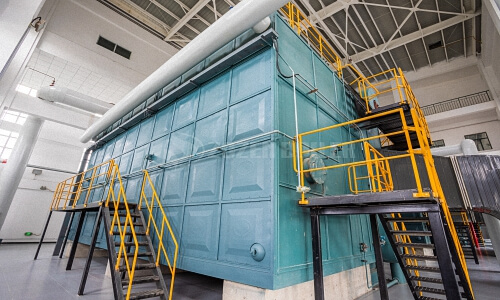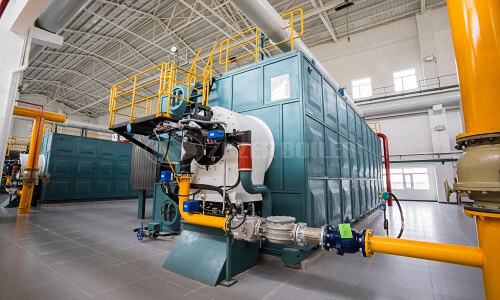# Biomass boiler efficiency calculation

2020-05-25

Biomass boiler Efficiency: Introduction and Methods of Calculation
It is a well known fact that the initial cost of biomass boiler is a small part of total costs associated with the boiler over its lifetime. In the operational life of a biomass boiler, major costs arise out of the fuel costs. Ensuring efficient operation of boiler is critical to optimize the fuel costs.

It is not always true that a biomass boiler will work at its rated efficiency. Almost all the times, it has been found that the biomass boiler operate at much lower than the rated efficiencies if proper efficiency monitoring is not done.

Biomass boiler Efficiency
Biomass boiler efficiency is a combined result of efficiencies of different components of a biomass boiler. A biomass boiler has many sub systems whose efficiency affects the overall biomass boiler efficiency. Couple of efficiencies which finally decide the biomass boiler efficiency are.

Combustion efficiency
Thermal efficiency
Apart from these efficiencies, there are some other losses which also play a role while deciding the biomass boiler efficiency and hence need to be considered while calculating the biomass boiler efficiency.

Combustion Efficiency
The combustion efficiency of a boiler is the indication of burner’s ability to burn fuel. The two parameters which determine the burner efficiency are unburnt fuel quantities in exhaust and excess oxygen levels in the exhaust. As the amount of excess air is increased, the quantity of unburnt fuel in the exhaust decreases. This results in lowering the unburnt fuel losses but elevating the enthalpy losses. Hence, it is quite important to maintain a balance between enthalpy losses and un burnt losses. Combustion efficiency also varies with the fuel being burnt. Combustion efficiency is higher for liquid and gaseous fuels than for solid fuels.Thermal Efficiency
The thermal efficiency of a biomass boiler specifies the effectiveness of the heat exchanger of the boiler which actually transfers the heat energy from fireside to water side. Thermal efficiency is badly affected by scale formation/soot formation on the boiler tubes.

Direct and Indirect Biomass boiler Efficiency
The overall biomass boiler efficiency depends on many more parameters apart from combustion and thermal efficiencies. These other parameters include ON-OFF losses, radiation losses, convection losses, blow down losses etc. In actual practice, two methods are commonly used to find out boiler efficiency, namely direct method and indirect method of efficiency calculation.

Direct efficiency
This method calculates biomass boiler efficiency by using the basic efficiency formula-

η=(Energy output)/(Energy input) X 100

In order to calculate boiler efficiency by this method, we divide the total energy output of a boiler by total energy input given to the boiler, multiplied by hundred.

Calculation of direct efficiency-

E= [Q (H-h)/q*GCV]*100

Where,

Q= Quantity of steam generated (kg/hr)

H= Enthalpy of steam (Kcal/kg)

h= Enthalpy of water (kcal/kg)

GCV= Gross calorific value of the fuel.Indirect Efficiency
The indirect efficiency of a boiler is calculated by finding out the individual losses taking place in a boiler and then subtracting the sum from 100%. This method involves finding out the magnitudes of all the measurable losses taking place in a boiler by separate measurements. All these losses are added and subtracted from 100% to find out the final efficiency. Blow down valve is kept closed during the procedure. This method should be implemented as per the norms provided in BS845 standards. The losses calculated include stack losses, radiation losses, blowdown losses etc.

Comparison of direct and indirect efficiency-
Both the methods of finding out boiler efficiency mentioned above have some advantages and some disadvantages associated with them. The greatest advantage of indirect method is that it also speaks about the sources of losses. By finding out indirect efficiency, one can come to know where the losses are increased and can be reduced. On the other hand, direct efficiency values are closer to reality as compared to indirect efficiency on account of uncovered losses such as radiation losses, ON-OFF losses etc. But direct efficiency can only tell us about the magnitude of overall loss. No information about individual losses and their magnitudes is conveyed from direct efficiency calculation. There always exists some difference in the values of direct and indirect efficiencies. Indirect efficiency is measured at a particular time whereas direct efficiency is measured over a period of time and hence, losses on account of fluctuating loads, boiler on-off etc. are also taken into consideration.

Real time efficiency monitoring
Biomass boiler efficiency does not remain fixed and large variations from ideal values take place during the course of operation. Shifting to real time efficiency monitoring can improve the biomass boiler efficiency significantly depending upon the boiler type and actual conditions at site. In nutshell, monitoring and maintaining the boiler efficiency for the overall operational.